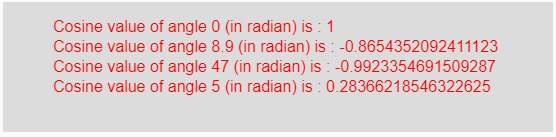# p5.js | cos() function

The cos() function in p5.js is used to calculate the cosine value of an angle (taken in radian) as input parameter of the function and returns the result between -1 to 1.

Syntax:

`cos(angle)`

Parameters: This function accepts a single parameter angle which is an angle in radian whose cosine value to be calculated.

Return Value: It returns the cosine value of an angle in radian taken as the input parameter.

Below program illustrates the cos() function in p5.js:

Example: This example uses cos() function to get cosine value of an angle in radian.

 `function` `setup() {  ` `  `  `    ``// Create Canvas of given size ` `    ``createCanvas(550, 130);  ` `}  ` `  `  `function` `draw() {  ` `      `  `    ``// Set the background color  ` `    ``background(220);  ` `      `  `    ``// Initialize the parameter with ` `    ``// angles in radian only ` `    ``let a = 0;  ` `    ``let b = 8.9;  ` `    ``let c = 47; ` `    ``let d = 5; ` `      `  `    ``// Call to cos() function  ` `    ``let v = cos(a); ` `    ``let w = cos(b); ` `    ``let x = cos(c); ` `    ``let y = cos(d); ` `      `  `    ``// Set the size of text  ` `    ``textSize(16);  ` `      `  `    ``// Set the text color  ` `    ``fill(color(``'red'``));  ` `    `  `    ``// Getting cosine value  ` `    ``text(``"Cosine value of angle 0 (in radian) is : "` `+ v, 50, 30); ` `    ``text(``"Cosine value of angle 8.9 (in radian) is : "` `+ w, 50, 50); ` `    ``text(``"Cosine value of angle 47 (in radian) is : "` `+ x, 50, 70); ` `    ``text(``"Cosine value of angle 5 (in radian) is : "` `+ y, 50, 90);      ` `}  `

Output:Note: In the above code, the input angle should be in radian. For converting degree angle into radian we can use the following formula:

`Angles_in_radian = (π/180)*angles_in_degree`

Reference: https://p5js.org/reference/#/p5/cos

My Personal Notes arrow_drop_upCheck out this Author's contributed articles.

If you like GeeksforGeeks and would like to contribute, you can also write an article using contribute.geeksforgeeks.org or mail your article to contribute@geeksforgeeks.org. See your article appearing on the GeeksforGeeks main page and help other Geeks.

Please Improve this article if you find anything incorrect by clicking on the "Improve Article" button below.

Improved By : ManasChhabra2

Article Tags :

Be the First to upvote.

Please write to us at contribute@geeksforgeeks.org to report any issue with the above content.